# Specifying a GLM in NiPy¶

In this tutorial we will discuss NiPy’s model and specification of a fMRI experiment.

This involves:

• an experimental model: a description of the experimental protocol (function of experimental time)

• a neuronal model: a model of how a particular neuron responds to the experimental protocol (function of the experimental model)

• a hemodynamic model: a model of the BOLD signal at a particular voxel, (function of the neuronal model)

## Experimental model¶

We first begin by describing typically encountered fMRI designs.

• Event-related categorical design, i.e. Face vs. Object

• Block categorical design

• Continuous stimuli, i.e. a rotating checkerboard

• Events with amplitudes, i.e. non-categorical values

• Events with random amplitudes

### Block categorical design¶

For block designs of the Face vs. Object type, we might also allow event durations, meaning that we show the subjects a Face for a period of, say, 0.5 seconds. We might represent this experiment graphically as follows,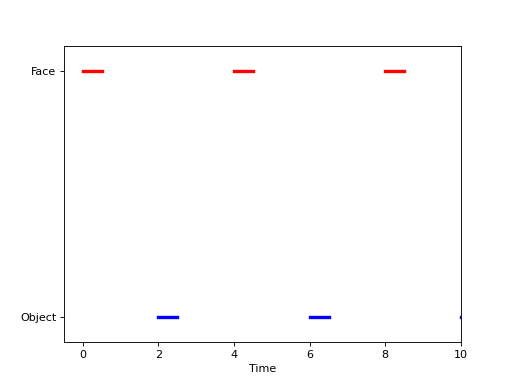and the intensity measure for the experiment could be expressed in terms of

\begin{split}\begin{aligned} E_a(t) &= E((-\infty,t], \{a\}) &= \sum_{t_j, \text{j odd}} \frac{1}{0.5} \int_{t_j}^ {\min(t_j+0.5, t)} \; ds \\ E_b(t) &= E((-\infty,t], \{b\}) &= \sum_{t_j, \text{j even}} \frac{1}{0.5} \int_{t_j}^ {\min(t_j+0.5, t)} \; ds \\ \end{aligned}\end{split}

The normalization chosen above ensures that each event has integral 1, that is a total of 1 “stimulus unit” is presented for each 0.5 second block. This may or may not be desirable, and could easily be changed.

### Continuous stimuli¶

Some experiments do not fit well into this “event-type” paradigm but are, rather, more continuous in nature. For instance, a rotating checkerboard, for which orientation, contrast, are functions of experiment time t. This experiment can be represented in terms of a state vector $$(O(t), C(t))$$. In this example we have set

import numpy as np

t = np.linspace(0,10,1000)
o = np.sin(2*np.pi*(t+1)) * np.exp(-t/10)
c = np.sin(2*np.pi*(t+0.2)/4) * np.exp(-t/12)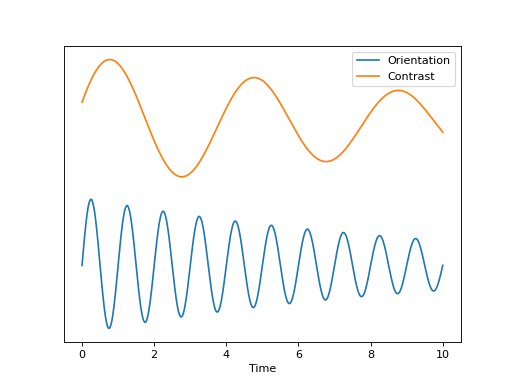The cumulative intensity measure for such an experiment might look like

$E([t_1, t_2], A) = \int_{t_1}^{t_2} \left(\int_A \; dc \; do\right) \; dt.$

In words, this reads as $$E([t_1,t_2],A)$$ is the amount of time in the interval $$[t_1,t_2]$$ for which the state vector $$(O(t), C(t))$$ was in the region $$A$$.

### Events with amplitudes¶

Another (event-related) experimental paradigm is one in which the event types have amplitudes, perhaps in a pain experiment with a heat stimulus, we might consider the temperature an amplitude. These amplitudes could be multi-valued. We might represent this parametric design mathematically as

$E = \sum_{j=1}^{10} \delta_{(t_j, a_j)},$

which is virtually identical to our description of the Face vs. Object experiment in face-object though the values $$a_j$$ are floats rather than labels. Graphically, this experiment might be represented as in this figure below.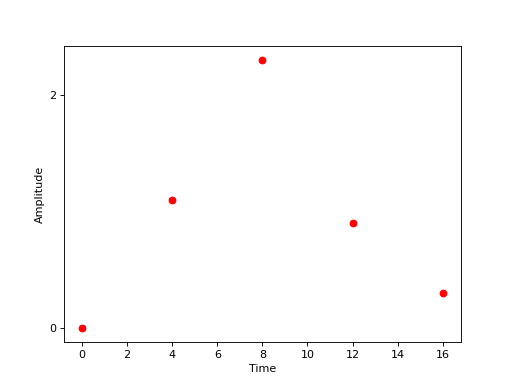### Events with random amplitudes¶

Another possible approach to specifying an experiment might be to deliver a randomly generated stimulus, say, uniformly distributed on some interval, at a set of prespecified event times.

We might represent this graphically as in the following figure.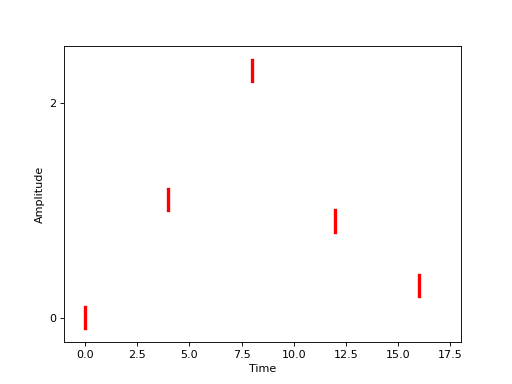Of course, the stimuli need not be randomly distributed over some interval, they could have fairly arbitrary distributions. Or, in the Face vs Object scenario, we could randomly present of one of the two types and the distribution at a particular event time $$t_j$$ would be represented by a probability $$P_j$$.

The cumulative intensity model for such an experiment might be

$E([t_1, t_2], A) = \sum_j 1_{[t_1, t_2]}(t_j) \int_A \; P_j(da)$

If the times were not prespecified but were themselves random, say uniform over intervals $$[u_j,v_j]$$, we might modify the cumulative intensity to be

$E([t_1, t_2], A) = \sum_j \int_{\max(u_j,t_1)}^{\min(v_j, t_2)} \int_A \; P_j(da) \; dt$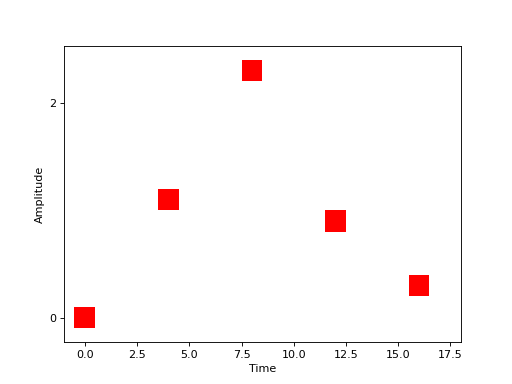# Neuronal model¶

The neuronal model is a model of the activity as a function of t at a neuron x given the experimental model $$E$$. It is most commonly expressed as some linear function of the experiment $$E$$. As with the experimental model, we prefer to start off by working with the cumulative neuronal activity, a measure on $$\mathbb{R}$$, though, ultimately we will work with the intensities in intensity.

Typically, the neuronal model with an experiment model $$E$$ has the form

$N([t_1,t_2]) = \int_{t_1}^{t_2}\int_V f(v,t) \; dE(v,t)$

Unlike the experimental model, which can look somewhat abstract, the neuronal model can be directly modeled. For example, take the standard Face vs. Object model face-object, in which case $$V=\{a,b\}$$ and we can set

$\begin{split}f(v,t) = \begin{cases} \beta_a & v = a \\ \beta_b & v = b \end{cases}\end{split}$

Thus, the cumulative neuronal model can be expressed as

from sympy import Symbol, Heaviside
t = Symbol('t')
ta = [0,4,8,12,16]
tb = [2,6,10,14,18]
ba = Symbol('ba')
bb = Symbol('bb')
fa = sum([Heaviside(t-_t) for _t in ta]) * ba
fb = sum([Heaviside(t-_t) for _t in tb]) * bb
N = fa+fb


Or, graphically, if we set $$\beta_a=1$$ and $$\beta_b=-2$$, as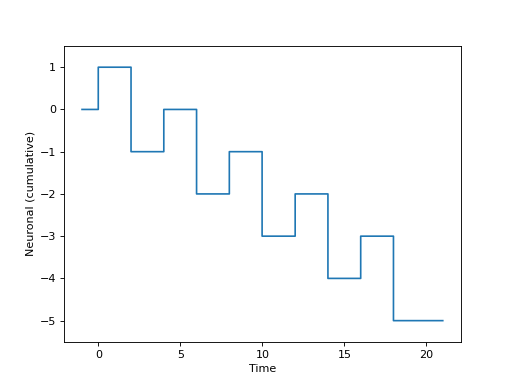In the block design, we might have the same form for the neuronal model (i.e. the same $$f$$ above), but the different experimental model $$E$$ yields

from sympy import Symbol, Piecewise
ta = [0,4,8,12,16]; tb = [2,6,10,14,18]
ba = Symbol('ba')
bb = Symbol('bb')
fa = sum([Piecewise((0, (t<_t)), ((t-_t)/0.5, (t<_t+0.5)), (1, (t >= _t+0.5))) for _t in ta])*ba
fb = sum([Piecewise((0, (t<_t)), ((t-_t)/0.5, (t<_t+0.5)), (1, (t >= _t+0.5))) for _t in tb])*bb
N = fa+fb


Or, graphically, if we set $$\beta_a=1$$ and $$\beta_b=-2$$, as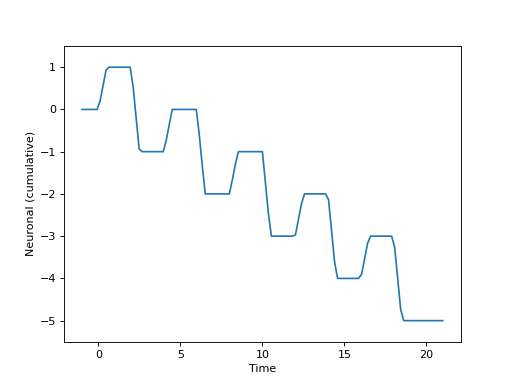The function $$f$$ above can be expressed as

$f(v,t) = \beta_a 1_{\{a\}}(v) + \beta_b 1_{\{b\}}(v) = \beta_a f_a(v,t) + \beta_b f_b(v,t)$

Hence, our typical neuronal model can be expressed as a sum

\begin{split}\begin{aligned} N([t_1,t_2]) &= \sum_i \beta_i \int_{t_1}^{t_2} \int_V f_i(v,t) \; dE(v,t) \\ &= \sum_i \beta_i \tilde{N}_{f_i}([t_1,t_2]) \end{aligned}\end{split}

for arbitrary functions $$\tilde{N}_{f_i}$$. Above, $$\tilde{N}_{f_i}$$ represents the stimulus contributed to $$N$$ from the function $$f_i$$. In the Face vs. Object example face-object, these cumulative intensities are related to the more common of neuronal model of intensities in terms of delta functions

$\frac{\partial}{\partial t} \tilde{N}_{f_a}(t) = \beta_a \sum_{t_i: \text{i odd}} \delta_{t_i}(t)$
from sympy import Symbol, Heaviside
ta = [0,4,8,12,16]
t = Symbol('t')
ba = Symbol('ba')
fa = sum([Heaviside(t-_t) for _t in ta]) * ba
print(fa.diff(t))

ba*(DiracDelta(t) + DiracDelta(t - 16) + DiracDelta(t - 12) + DiracDelta(t - 8) + DiracDelta(t - 4))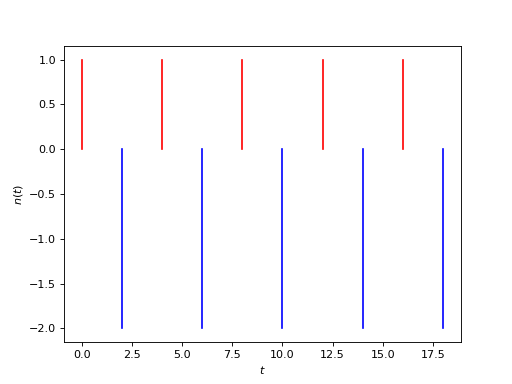## Convolution¶

In our continuous example above, with a periodic orientation and contrast, we might take

\begin{split}\begin{aligned} f_O(t,(o,c)) &= o \\ f_O(t,(o,c)) &= c \\ \end{aligned}\end{split}

yielding a neuronal model

$N([t_1,t_2]) = \beta_{O} O(t) + \beta_{C} C(t)$

We might also want to allow a delay in the neuronal model

$N^{\text{delay}}([t_1,t_2]) = \beta_{O} O(t-\tau_O) + \beta_{C} C(t-\tau_C).$

This delay can be represented mathematically in terms of convolution (of measures)

$N^{\text{delay}}([t_1,t_2]) = \left(\tilde{N}_{f_O} * \delta_{-\tau_O}\right)([t_1, t_2]) +\left(\tilde{N}_{f_C} * \delta_{-\tau_C}\right)([t_1, t_2])$

Another model that uses convolution is the Face vs. Object one in which the neuronal signal is attenuated with an exponential decay at time scale $$\tau$$

$D([t_1, t_2]) = \int_{\max(t_1,0)}^{t_2} \tau e^{-\tau t} \; dt$

yielding

$N^{\text{decay}}([t_1,t_2]) = (N * D)[t_1, t_2]$

# Events with amplitudes¶

We described a model above event-amplitude with events that each have a continuous value $$a$$ attached to them. In terms of a neuronal model, it seems reasonable to suppose that the (cumulative) neuronal activity is related to some function, perhaps expressed as a polynomial $$h(a)=\sum_j \beta_j a^j$$ yielding a neuronal model

$N([t_1, t_2]) = \sum_j \beta_j \tilde{N}_{a^j}([t_1, t_2])$

## Hemodynamic model¶

The hemodynamic model is a model for the BOLD signal, expressed as some function of the neuronal model. The most common hemodynamic model is just the convolution of the neuronal model with some hemodynamic response function, $$HRF$$

\begin{split}\begin{aligned} HRF((-\infty,t]) &= \int_{-\infty}^t h_{can}(s) \; ds \\ H([t_1,t_2]) & = (N * HRF)[t_1,t_2] \end{aligned}\end{split}

The canonical one is a difference of two Gamma densities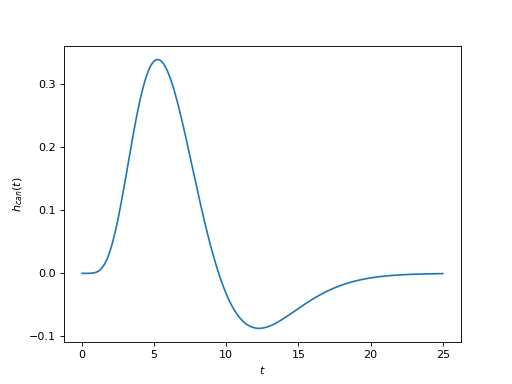## Intensities¶

Hemodynamic models are, as mentioned above, most commonly expressed in terms of instantaneous intensities rather than cumulative intensities. Define

$n(t) = \frac{\partial}{\partial t} N((-\infty,t]).$

The simple model above can then be written as

$h(t) = \frac{\partial}{\partial t}(N * HRF)(t) = \int_{-\infty}^{\infty} n(t-s) h_{can}(s) \; ds.$

In the Face vs. Object experiment, the integrals above can be evaluated explicitly because $$n(t)$$ is a sum of delta functions

$n(t) = \beta_a \sum_{t_i: \text{i odd}} \delta_{t_i}(t) + \beta_b \sum_{t_i: \text{i even}} \delta_{t_i}(t)$

In this experiment we may want to allow different hemodynamic response functions within each group, say $$h_a$$ within group $$a$$ and $$h_b$$ within group $$b$$. This yields a hemodynamic model

$h(t) = \beta_a \sum_{t_i: \text{i odd}} h_a(t-t_i) + \beta_b \sum_{t_i: \text{i even}} h_b(t-t_i)$
from nipy.modalities.fmri import hrf

ta = [0,4,8,12,16]; tb = [2,6,10,14,18]
ba = 1; bb = -2
na = ba * sum([hrf.glover(hrf.T - t) for t in ta])
nb = bb * sum([hrf.afni(hrf.T - t) for t in tb])
n = na + nb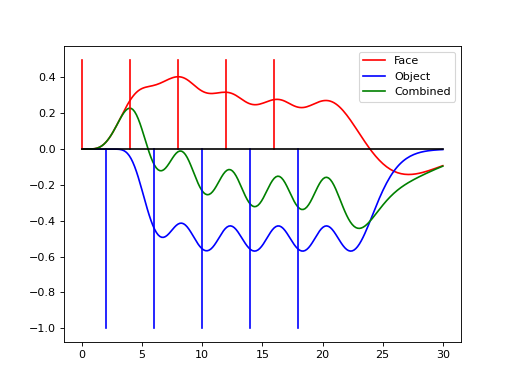Applying the simple model to the events with amplitude model and the canonical HRF yields a hemodynamic model

$h(t) = \sum_{i,j} \beta_j a_i^j h_{can}(t-t_i)$
import numpy as np
from nipy.modalities.fmri.utils import events, Symbol

a = Symbol('a')
b = np.linspace(0,50,6)
amp = b*([-1,1]*3)
d = events(b, amplitudes=amp, g=a+0.5*a**2, f=hrf.glover)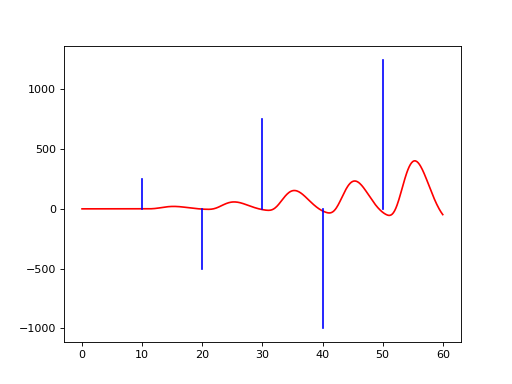## Derivative information¶

In cases where the neuronal model has more than one derivative, such as the continuous stimuli continuous-stimuli example, we might model the hemodynamic response using the higher derivatives as well. For example

$h(t) = \beta_{O,0} \tilde{n}_{f_O}(t) + \beta_{O,1} \frac{\partial}{\partial t}\tilde{n}_{f_O}(t) + \beta_{C,0} \tilde{n}_{f_C}(t) + \beta_{C,1} \frac{\partial} {\partial t}\tilde{n}_{f_C}(t)$

where

\begin{split}\begin{aligned} \tilde{n}_f(t) &= \frac{\partial}{\partial t} \tilde{N}_f((-\infty,t]) \\ &= \frac{\partial}{\partial t} \left( \int_{-\infty}^t \int_V f(v,t) \; dE(v,t) \right) \end{aligned}\end{split}

# Design matrix¶

In a typical GLM analysis, we will compare the observed BOLD signal $$B(t)$$ at some fixed voxel $$x$$, observed at time points $$(s_1, \dots, s_n)$$, to a hemodynamic response model. For instance, in the Face vs. Object model, using the canonical HRF

$B(t) = \beta_a \sum_{t_i: \text{i odd}} h_{can}(t-t_i) + \beta_b \sum_{t_i: \text{i even}} h_{can}(t-t_i) + \epsilon(t)$

where $$\epsilon(t)$$ is the correlated noise in the BOLD data.

Because the BOLD is modeled as linear in $$(\beta_a,\beta_b)$$ this fits into a multiple linear regression model setting, typically written as

$Y_{n \times 1} = X_{n \times p} \beta_{p \times 1} + \epsilon_{n \times 1}$

In order to fit the regression model, we must find the matrix $$X$$. This is just the derivative of the model of the mean of $$B$$ with respect to the parameters to be estimated. Setting $$(\beta_1, \beta_2)=(\beta_a, \beta_b)$$

$X_{ij} = \frac{\partial}{\partial \beta_j} \left(\beta_1 \sum_{t_k: \text{k odd}} h_{can}(s_i-t_k) + \beta_b \sum_{t_k: \text{k even}} h_{can}(s_i-t_k) \right)$

## Drift¶

We sometimes include a natural spline model of the drift here.

This changes the design matrix by adding more columns, one for each function in our model of the drift. In general, starting from some model of the mean the design matrix is the derivative of the model of the mean, differentiated with respect to all parameters to be estimated (in some fixed order).

## Nonlinear example¶

The delayed continuous stimuli example above is an example of a nonlinear function of the mean that is nonlinear in some parameters, $$(\tau_O, \tau_C)$$.

# Formula objects¶

This experience of building the model can often be simplified, using what is known in :ref:R as formula objects. NiPy has implemented a formula object that is similar to R’s, but differs in some important respects. See nipy.algorithms.statistics.formula.